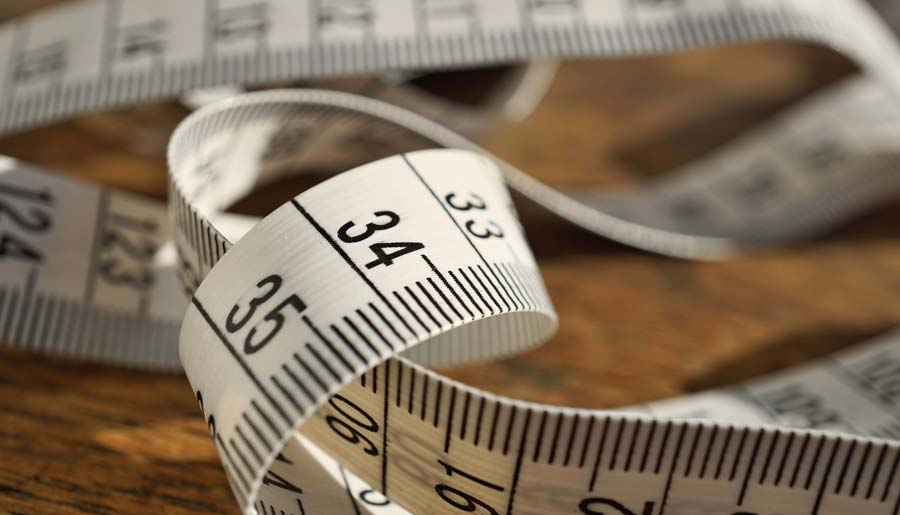# How Many Feet Are In a Meter/Metre?

| Last update: 31 March 2021

Today we're going to look at the conversion of meters to feet. Both of these are units of length. The meter is the base unit in the International System of Units and is equal to the distance travelled by light through a vacuum in 1/299,792,458 seconds. The foot (plural: feet) is an imperial unit originating from the length of the human foot.You may have noticed my use of the spelling 'meter.' A number of people over the years have written to me to say that I am spelling the unit incorrectly by adopting 'meter' as the default spelling in many of my converters and articles. Use of the word 'meter' in the UK is commonly reserved for describing an instrument that measures the flow of electricity, gas, water etc. However, as far as the unit is concerned, there are actually two spellings for the meter - the international spelling of 'metre' and the US spelling of 'meter.'

There are 3.280839895 feet in a meter. (rounded to 9 decimal places)

1 meter = 3.280839895 feet

To assist you with converting meters to feet, I have included a chart and a converter below. I've also created a similar article discussing how many feet there are in a mile.

## Meters to feet chart

In the chart below, the figures for feet are rounded to 4 decimal places and the figures for feet and inches are rounded to 2 decimal places. For a specific number conversion, please use the converter tool underneath this chart.

Meters Feet Feet and Inches
1 3.2808 ft 3 ft, 3.37 in
2 6.5617 ft 6 ft, 6.74 in
3 9.8425 ft 9 ft, 10.11 in
4 13.1234 ft 13 ft, 1.48 in
5 16.4042 ft 16 ft, 4.85 in
6 19.685 ft 19 ft, 8.22 in
7 22.9659 ft 22 ft, 11.59 in
8 26.2467 ft 26 ft, 2.96 in
9 29.5276 ft 29 ft, 6.33 in
10 32.8084 ft 32 ft, 9.7 in

## Meters to feet converter

If you're looking for a pain-free method of converting between meters and feet right here and now then you can use our handy converter, below. As an alternative, should you wish to convert between meters, feet AND inches then please try the converter for meters to feet and inches or the one for feet to meters.

My website provides a comprehensive list of online conversion tools. So, should you wish to convert between meters, feet, inches, and any other units of length or distance, give the length and distance converter a try.

Steve Reid has written to me to suggest a simple way of estimating m to ft (and vice versa) in your head. Here's the concept:

• m to ft: multiply by 10, divide by 3, round down by 16 parts per thousand.
• ft to m: multiply by 3, divide by 10, round up by 16 per thousand.

Example: Ben Nevis is 1344m high. How high is it in feet?

1344 × 10 = 13440
13440 / 3 = 4480
Round down = 16 * 4.5 (rounded) = 72
4480 - 72 = 4408

Gives us a total of 4,408 feet. Using the converter, we find that 1,344m is actually 4,409ft. So, we're 1 foot out, but as a rough estimate we're good.